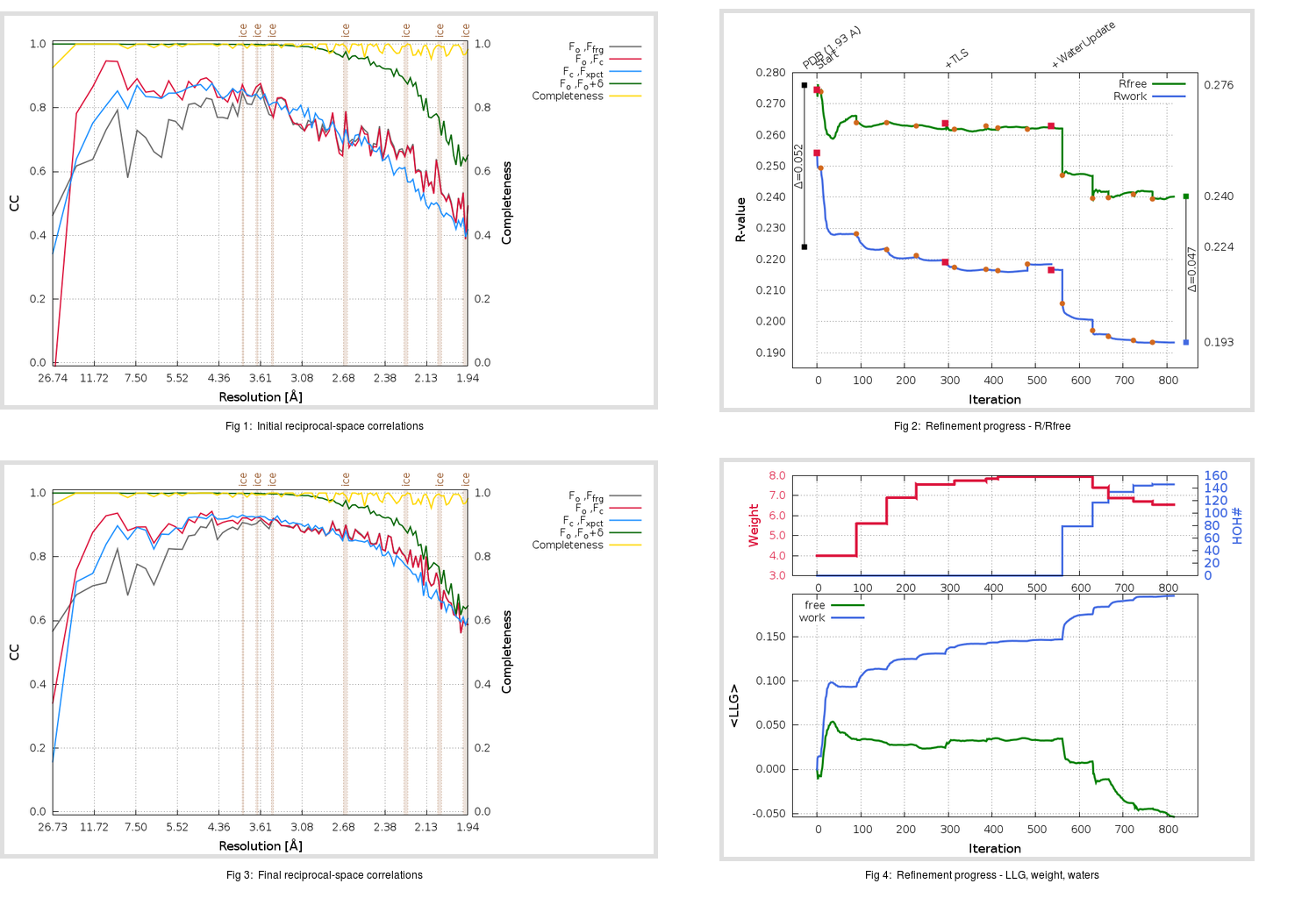Content:

```    Diffraction limits & principal axes of ellipsoid fitted to diffraction cut-off surface:
1.972         0.9747   0.0000   0.2237       0.798 a* - 0.603 c*
1.848         0.0000   1.0000   0.0000       b*
1.863        -0.2237   0.0000   0.9747      -0.425 a* + 0.905 c*
```

## Deposited

` `
 Date deposited Date data collection Resolution R, Rfree 20200303 20200226 1.93 0.2220 0.2760

Molprobity (CCP4 7.0 version) summary:

```Ramachandran outliers =   0.33 %
favored =  96.36 %
Rotamer outliers      =   1.13 %
C-beta deviations     =     0
Clashscore            =   6.71
RMS(bonds)            =   0.0131
RMS(angles)           =   1.83
MolProbity score      =   1.65
Resolution            =   1.93
R-work                =   0.2220
R-free                =   0.2760
```

```Number of waters      =    99

<B> (all atoms) =   35.46 ( sd =   13.31 ) for       2511 non-hydrogen atoms
<B>   (protein) =   33.83 ( sd =   10.06 ) for       2382 non-hydrogen atoms
<B>     (water) =   58.38 ( sd =   17.21 ) for         99 non-hydrogen atoms
<B>    (others) =   86.99 ( sd =   32.72 ) for         30 non-hydrogen atoms

B min/max       (all non-hydrogen atoms) =   19.66 /  142.95
B min/max   (protein non-hydrogen atoms) =   19.66 /   82.47
B min/max     (water non-hydrogen atoms) =   31.54 /  119.37
B min/max     (other non-hydrogen atoms) =   52.32 /  142.95
```

## BUSTER (re-)refinement

` `

Molprobity (CCP4 7.0 version) summary:

```Ramachandran outliers =   0.33 %
favored =  98.68 %
Rotamer outliers      =   0.38 %
C-beta deviations     =     0
Clashscore            =   3.83
RMS(bonds)            =   0.0120
RMS(angles)           =   1.61
MolProbity score      =   1.17
Resolution            =   1.93
R-work                =   0.1933
R-free                =   0.2403
```

```Number of waters      =   146

<B> (all atoms) =   33.85 ( sd =    8.98 ) for       2523 non-hydrogen atoms
<B>   (protein) =   33.11 ( sd =    8.23 ) for       2347 non-hydrogen atoms
<B>     (water) =   40.50 ( sd =    9.26 ) for        146 non-hydrogen atoms
<B>    (others) =   59.59 ( sd =   12.36 ) for         30 non-hydrogen atoms

B min/max       (all non-hydrogen atoms) =   19.82 /   78.25
B min/max   (protein non-hydrogen atoms) =   19.82 /   73.66
B min/max     (water non-hydrogen atoms) =   21.04 /   68.51
B min/max     (other non-hydrogen atoms) =   37.22 /   78.25
```

Refinement progression:Results:

` `
 File Remark 5R80_aB_refine_C2.01_03_refine.pdb.gz exact refinement commands are in header 5R80_aB_refine_C2.01_03_refine.mtz.gz including original deposited data and several re-refinement map coefficients 5R80_aB_refine_C2.01_03_BUSTER_model.cif.gz including any non-standard compound restraints 5R80_aB_refine_C2.01_03_BUSTER_refln.cif.gz